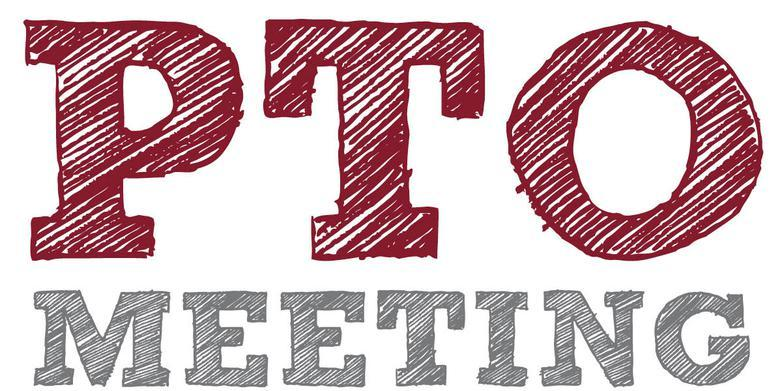/* styles */ Hi DC Community!

# The Desert Cove eNews is sent on a MONTHLY basis.

If you do not wish to receive the Desert Cove PTO eNews,
please click 'Unsubscribe' at the bottom of this emailMARCH 2019

▪ Mar 12: PTO Meeting @ 6PM in Library
▪ Mar 13: UPC Meeting
▪ Mar 13: Teacher/Staff Appreciation Nomination Deadline
▪ Mar 14: K-3 Field Day (VOLUNTEERS NEEDED)
▪ Mar 15: Breakfast with Buddies (VOLUNTEERS NEEDED)
▪ Mar 18-22: SPRING BREAK
▪ Mar 25: Yearbook Pre-Order DEADLINE!
▪ Mar 27: DC Spirit Night @ PDQ
 ▪ Mar 12: PTO Meeting @ 6PM in Library
 ▪ Mar 13: UPC Meeting
 ▪ Mar 14: K-3 Field Day (VOLUNTEERS NEEDED)
 ▪ Mar 15: Breakfast with Buddies (VOLUNTEERS NEEDED)
 ▪ Mar 18-22: SPRING BREAK
 ▪ Mar 25: Yearbook Pre-Order DEADLINE!
 ▪ Mar 27: DC Spirit Night @ PDQ

 table div table+table+table+table+table+table div table{width:100%;padding:0}table div table+table+table+table+table+table div table img{width:96.23%;padding:0;float:none}table div table+table+table+table+table+table div table td{width:100%;padding:0 1.88% 18px}/* styles */table.module-6{width:99.06%;padding:0}table div table+table+table+table+table+table+table div table{width:99.06%;float:none;margin-left:auto;margin-right:auto;padding:0}table div table+table+table+table+table+table+table div table a{border:0 none;text-decoration:none}table div table+table+table+table+table+table+table div table img{width:100%!important;border:0 none;text-decoration:none}table div table+table+table+table+table+table+table div table td{width:100%;padding:0}/* styles */

# The Desert Cove PTO communicates information in many ways to our DC Families!Pick one or more that work best for your family!Click the image below for more details!

 table div table+table+table+table+table+table+table+table+table div table td,table.module-8{width:100%;padding:0}table div table+table+table+table+table+table+table+table+table div table{width:100%;float:none;margin-left:auto;margin-right:auto;padding:0}table div table+table+table+table+table+table+table+table+table div table a{border:0 none;text-decoration:none}table div table+table+table+table+table+table+table+table+table div table img{width:100%!important;border:0 none;text-decoration:none}/* styles */
 table div table+table+table+table+table+table+table+table+table+table div table{width:100%;padding:0}table div table+table+table+table+table+table+table+table+table+table div table img{width:96.23%;padding:0;float:none}table div table+table+table+table+table+table+table+table+table+table div table td{width:100%;padding:0 1.88% 18px}/* styles */## MAR 12: PTO MEETING# FREE Pizza & Childcare provided!

Can't make the meeting? No worries, Meeting Minutes are posted on our website:

www.desertcovepto.org/meetings

 table div table+table+table+table+table+table+table+table+table+table+table+table+table div table{width:100%;padding:0}table div table+table+table+table+table+table+table+table+table+table+table+table+table div table img{width:96.23%;padding:0;float:none}table div table+table+table+table+table+table+table+table+table+table+table+table+table div table td{width:100%;padding:0 1.88% 18px}/* styles */## Mar 15: BREAKFAST WITH BUDDIES7am-7:45am in the Cafeteria# Join us for our annual Breakfast with Buddies event brought to you by theDesert Cove PTO!

## This a DC Community Event where students can bring ANYspecial adult with them for a fun breakfast at the school!A FEW MORE VOLUNTEERS ARE NEEDED! (click below)

 table div table+table+table+table+table+table+table+table+table+table+table+table+table+table+table+table+table div table{width:100%;padding:0}table div table+table+table+table+table+table+table+table+table+table+table+table+table+table+table+table+table div table img{width:96.23%;padding:0;float:none}table div table+table+table+table+table+table+table+table+table+table+table+table+table+table+table+table+table div table td{width:100%;padding:0 1.88% 18px}/* styles */## MAR 25: YEARBOOK PRE-ORDER DEADLINE# YEARBOOK PRE-ORDERS ON SALE NOW!

## Pre-order your Yearbook from now through March 25th!We aren't going to be ordering a large quantity of books to sell on campus this year, so make sure to pre-order yours!

NOTE: Pre-ordering is the ONLY way to get the cover of your child's yearbook personalized!

Pre-order Yearbook is \$20
Pre-Order Personalized \$25
Yearbook in May (if not sold out in presale) \$25 CASH ONLY (not personalized)

 table div table+table+table+table+table+table+table+table+table+table+table+table+table+table+table+table+table+table+table+table+table div table{width:100%;padding:0}table div table+table+table+table+table+table+table+table+table+table+table+table+table+table+table+table+table+table+table+table+table div table img{width:96.23%;padding:0;float:none}table div table+table+table+table+table+table+table+table+table+table+table+table+table+table+table+table+table+table+table+table+table div table td{width:100%;padding:0 1.88% 18px}/* styles */## MAR 27: DC SPIRIT NIGHT @ PDQ4514 E. Cactus Road5pm - 8pm

 table div table+table+table+table+table+table+table+table+table+table+table+table+table+table+table+table+table+table+table+table+table+table+table div table td,table.module-22{width:100%;padding:0}table div table+table+table+table+table+table+table+table+table+table+table+table+table+table+table+table+table+table+table+table+table+table+table div table{width:100%;float:none;margin-left:auto;margin-right:auto;padding:0}table div table+table+table+table+table+table+table+table+table+table+table+table+table+table+table+table+table+table+table+table+table+table+table div table a{border:0 none;text-decoration:none}table div table+table+table+table+table+table+table+table+table+table+table+table+table+table+table+table+table+table+table+table+table+table+table div table img{width:100%!important;border:0 none;text-decoration:none}/* styles */
 table div table+table+table+table+table+table+table+table+table+table+table+table+table+table+table+table+table+table+table+table+table+table+table+table+table div table{width:100%;padding:0}table div table+table+table+table+table+table+table+table+table+table+table+table+table+table+table+table+table+table+table+table+table+table+table+table+table div table img{width:96.23%;padding:0;float:none}table div table+table+table+table+table+table+table+table+table+table+table+table+table+table+table+table+table+table+table+table+table+table+table+table+table div table td{width:100%;padding:0 1.88% 18px}/* styles */We have created a page on the PTO website where you can access the following communications:

Desert Cove PTO eNews archives
Desert Cove Principal News
PV School District eNews
United Parent Council eNews